Browse by Topic
Related Topics

# New Wolfram Books: Adventures, Lessons and Computations to Spark Your Curiosity

It’s been another big year of exploration with the Wolfram Language. CEO Stephen Wolfram’s new book takes us on a tour of his computational adventures throughout the years. We’re also excited to introduce a Spanish-language version of the popular An Elementary Introduction to the Wolfram Language, as well as books to enhance the mathematics and engineering curricula. There’s something new for everyone, from students to lifelong adventurers, to discover with the Wolfram Language. Just in time for the holidays, find the perfect read for those who love learning new things—including yourself!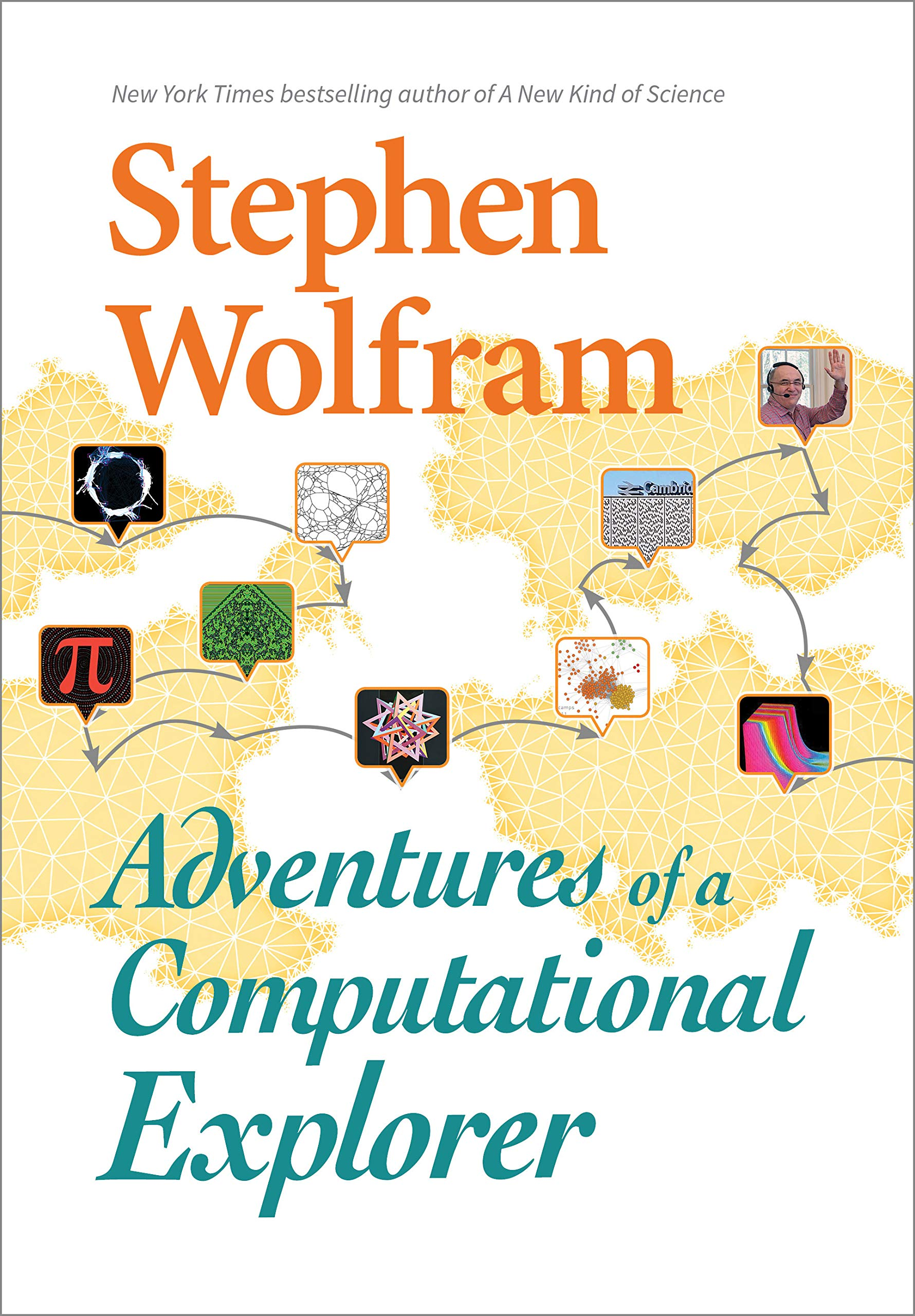## Adventures of a Computational Explorer

Join Stephen Wolfram as he brings the reader along on some of his most surprising and engaging intellectual adventures, showcasing his own signature way of thinking about an impressive range of subjects. From science consulting for a Hollywood movie, solving problems of AI ethics, hunting for the source of an unusual polyhedron, communicating with extraterrestrials, to finding the fundamental theory of physics and exploring the digits of pi, this lively book of essays captures the infectious energy and curiosity of one of the great pioneers of the computational world.

## Una introducción elemental a Wolfram Language (Spanish)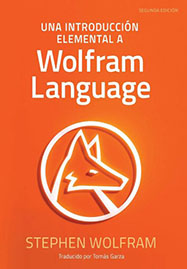¡Ahora en español por primera vez! Stephen Wolfram’s introduction to modern computational thinking is now available in Spanish, bringing the leading-edge computation of the Wolfram Language to an even larger audience. The Wolfram Language scales from a single line of easy-to-understand, interactive code to million-line production systems. The book does not assume any programming knowledge, making it suitable for students of all levels, as well as anyone interested in learning the latest technology and the world’s only large-scale computational language. You can also find the second edition of the English version here.

## Máquinas y mecanismos, implementación con Wolfram Mathematica (Spanish)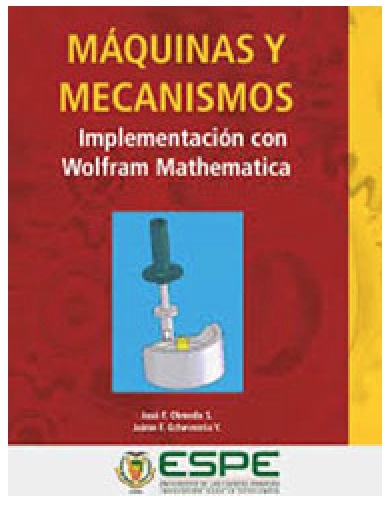Look inside the machines that power our civilization and provide innumerable goods and services, and you will find mechanisms at the core. This book is designed to supplement the existing curriculum and lab work by students. Building on the foundations from the classroom, Máquinas y mecanismos, implementación con Wolfram Mathematica enhances this branch of engineering for both sides of the instructor’s desk. Students engage more deeply with the material; examples and small Wolfram Language programs embedded within the text encourage the dynamic exploration of the behavior of any linkage. The Manipulate function in the Wolfram Language allows students to easily examine effects produced by variations in the lengths of the links or their relationships, the linear and angular speeds and accelerations of the input link, and the inertial parameters in the behavior of a mechanism.

## Mathematical Analysis and Instrumental Methods for Solving Problems (Russian)

### Book 1  |  Book 2

Compiled into two books, materials from the Mathematical Analysis course by authors Vladimir Grigorievich Chirsky and Kirill Yuryevich Shilin bring theoretical mathematics closer to instrumental methods. Sit in on the first and second semesters of the course (originally taught at the Department of Economics of the Institute of Economics, Mathematics and Information Technology, RANEPA), with each book chapter thoughtfully divided into four sections: 1) basic definitions, statement of theorems and simple proofs; 2) evidence of theorems and more in-depth readings; 3) solutions to common problems; and 4) how to solve the problems using Mathematica.

## Discrete Mathematics: Learning to Program in Wolfram Mathematica (Russian)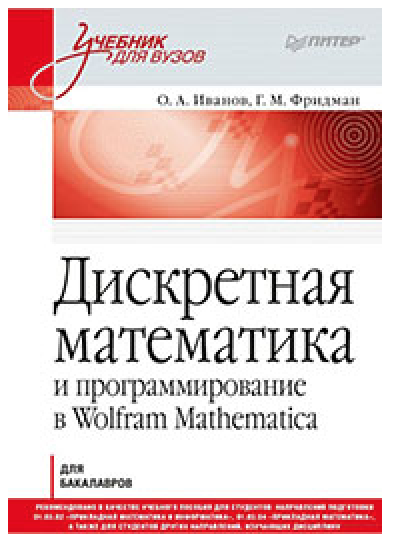Discrete mathematics is as essential for a programmer as an applied mathematician, but many students struggle with the subject. Discrete Mathematics: Learning to Program in Wolfram Mathematica combines an introduction to the main concepts and methods of discrete mathematics with the basics of programming in Mathematica. This book takes you through a range of theoretical and applied discrete mathematics, including combinatorics and enumerative combinatorics, data structures such as binary heaps and binary search trees, sorting algorithms, and operations in residue rings and modern encryption methods. Twelve Mathematica “programming lessons” show the actual code to implement all the algorithms introduced throughout the chapters, making this book a valuable resource for students, university professors and anyone interested in learning how to program in Mathematica.

## The Student’s Introduction to Mathematica and the Wolfram Language, Third Edition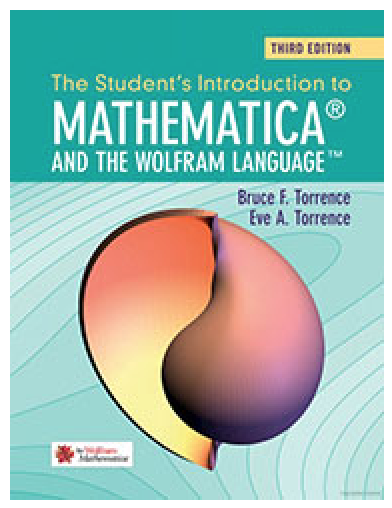This compact, new edition of The Student’s Introduction to Mathematica and the Wolfram Language is an excellent companion to the standard mathematics curriculum. A brief introduction to the aspects of the Mathematica software program most useful to students, this third edition includes significant updated material to account for the latest developments in the Wolfram Language. As a result, this book also serves as a great tutorial for former students looking to learn about new features such as natural language queries, 3D printing and computational geometry, as well as the vast stores of real-world data now integrated through the cloud.